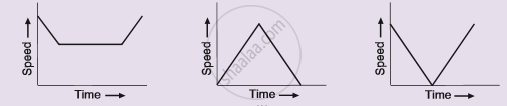Share

# Three Speed-time Graphs Are Given Below : - CBSE Class 9 - Science

ConceptGraphical Representation of Motion - Velocity-time Graphs

#### Question

Three speed-time graphs are given below :Which graph represents the case of:
(i) a cricket ball thrown vertically upwards and returning to the hands of the thrower ?
(ii) a trolley decelerating to a constant speed and then accelerating uniformly ?

#### Solution

(i) Graph (c) represents a cricket ball thrown vertically upwards and returning to the hands of the thrower.
(ii) Graph (a) represents a trolley decelerating to a constant speed and then accelerating uniformly.

Is there an error in this question or solution?

#### APPEARS IN

Solution Three Speed-time Graphs Are Given Below : Concept: Graphical Representation of Motion - Velocity-time Graphs.
S